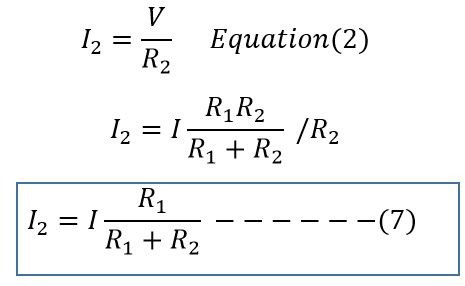Search

# Current Divider Rule: What is it? Formula, Derivation & Examples

When current source is connected to the circuit elements which are in parallel, the total current flowing in the circuit gets divided in each element according to resistance or impedance of the circuit element. Thus, the current is divided in all the branches. The voltage across each branch element remains the same because these elements are connected in the parallel.

## Current Divider Formula Derivation

The current division or current divider rule can be well understood with following explanation.The current through R1 and R2 Resistance can be calculated using Ohm’s law;

Current through resistance R1

Current through resistance R1Current through resistance R2Total equivalent resistance of the parallel circuit is RTotal current flowing in the circuit is I ;Putting the value of V in equation (1) we get;Putting the value of V in equation (2) we get;## Current Divider Formula

Thus, the current in the parallel branch is equal to the ratio of opposite branch resistance to the total resistance multiplied by the total circuit current. The current divider formula is as given below.### Illustrative Example on Current Divider:

Two resistances 50Ω and 100Ω is connected in parallel with a current source of 50A. Find the current flow through each resistor.Current through 50 Ω resistance is I1Current through 100 Ω resistance is I2Note:

Current divider rule is used when two or more circuit elements are connected in parallel with a current source.

When the two or more circuit elements are connected in parallel with voltage source, the current in the each branch can be calculated by simply applying the Ohm’s law because voltage across each circuit element is the same and by dividing the voltage with branch resistance, the current in the branch can be easily calculated.